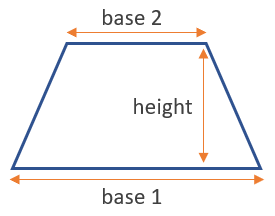# Area of a Trapezoid Calculator

Use this calculator to easily calculate the area of a trapezoid given its bases and height.

Share calculator:

Embed this tool:
get code

## Area of a trapezoid formula

The formula for the area of a trapezoid is (base 1 + base 2) / 2 x height, as seen in the figure below:The calculation essentially relies on the fact a trapezoid's area can be equated to that of a rectangle: (base 1 + base 2) / 2 is actually the width of a rectangle with an equivalent area. In order to use our area of a trapezoid calculator, you need to take three measurements, all in the same units (convert as necessary).

The result is always in the length unit used - but squared. So, if you measured in feet, the result will be in square feet. If you measured in centimeters, the result will be in square centimeters, and so on for square inches, yards, miles, as well as square meters, kilometers...

## Area of a Trapezoid calculation

To make the calculation, first, measure the two bases. Then, build the height using a right angle with a tip at any of the bases and using that base as one of its arms. Measure the height and make the necessary metric conversions until all three lengths are in the same units. Then apply the formula above or use our area of a trapezoid calculator online to save time and have a higher chance that the result is error-free (bad input will certainly result in bad output).

## Example: find the area of a trapezoid

As suggested by the equation above, there are three measurements needed to calculate the area of any trapezoid: the lengths of its bases and its height. Then it is just a matter of plugging in the values into the formula. For example, if one base is 6 cm long, the other is 10 cm long and the height is 4 cm, the area is then (6 + 10) / 2 x 4 = 16 / 2 x 4 = 8 x 4 = 32 cm2 (32 square centimeters).

#### Cite this calculator & page

If you'd like to cite this online calculator resource and information as provided on the page, you can use the following citation:
Georgiev G.Z., "Area of a Trapezoid Calculator", [online] Available at: https://www.gigacalculator.com/calculators/area-of-trapezoid-calculator.php URL [Accessed Date: 27 Mar, 2023].